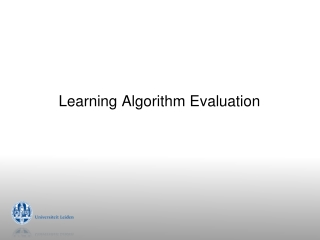DownloadDownload PresentationLearning Algorithm Evaluation

# Learning Algorithm Evaluation

Download Presentation## Learning Algorithm Evaluation

- - - - - - - - - - - - - - - - - - - - - - - - - - - E N D - - - - - - - - - - - - - - - - - - - - - - - - - - -
##### Presentation Transcript

1. Learning Algorithm Evaluation

2. Introduction

3. Overfitting

4. Overfitting A model should perform well on unseen data drawn from the same distribution

5. Evaluation Rule #1 Never evaluate on training data

6. Train and Test Step 1: Randomly split data into training and test set (e.g. 2/3-1/3) a.k.a. holdout set

7. Train and Test Step 2: Train model on training data

8. Train and Test Step 3: Evaluate model on test data

9. Train and Test Quiz: Can I retry with other parameter settings?

10. Evaluation Rule #1 Never evaluate on training data Rule #2 Never train on test data (that includes parameter setting or feature selection)

11. Test data leakage • Never use test data to create the classifier • Separating train/test can be tricky: e.g. social network • Proper procedure uses three sets • training set: train models • validation set: optimize algorithm parameters • test set: evaluate final model

12. Train and Test Step 4: Optimize parameters on separate validation set validation testing

13. Making the most of the data • Once evaluation is complete, all the data can be used to build the final classifier • Trade-off: performance  evaluation accuracy • More training data, better model (but returns diminish) • More test data, more accurate error estimate

14. Train and Test Step 5: Build final model on ALL data (more data, better model)

15. Cross-Validation

16. k-fold Cross-validation • Split data (stratified) in k folds • Use (k-1) for training, 1 for testing • Repeat k times • Average results Original Fold 1 Fold 2 Fold 3 train test

17. Cross-validation • Standard method: • Stratified ten-fold cross-validation • 10? Enough to reduce sampling bias • Experimentally determined

18. Leave-One-Out Cross-validation • A particular form of cross-validation: • #folds = #examples • n examples, build classifier n times • Makes best use of the data, no sampling bias • Computationally expensive Original Fold 1 Fold 100 100 ………

19. ROC Analysis

20. ROC Analysis • Stands for “Receiver Operating Characteristic” • From signal processing: trade-off between hit rate and false alarm rate over noisy channel • Compute FPR, TPR and plot them in ROC space • Every classifier is a point in ROC space • For probabilistic algorithms • Collect many points by varying prediction threshold

21. Confusion Matrix actual - + TP FP + true positive false positive predicted TN FN - false negative true negative FP+TN TP+FN TPRate: FPRate:

22. ROC space J48 parameters fitted J48 PRISM classifiers

23. Different Costs • In practice, TP and FN errors incur different costs • Examples: • Promotional mailing: will X buy the product? • Loan decisions: approve mortgage for X? • Medical diagnostic tests: does X have leukemia? • Add cost matrix to evaluation that weights TP, FP,...

24. equal costs skewed costs ROC Space and Costs

25. Statistical Significance

26. Comparing data mining schemes • Which of two learning algorithms performs better? • Note: this is domain dependent! • Obvious way: compare 10-fold CV estimates • Problem: variance in estimate • Variance can be reduced using repeated CV • However, we still don’t know whether results are reliable

27. Significance tests • Significance tests tell us how confident we can be that there really is a difference • Null hypothesis: there is no “real” difference • Alternative hypothesis: there is a difference • A significance test measures how much evidence there is in favor of rejecting the null hypothesis • E.g. 10 cross-validation scores: B better than A? mean A mean B P(perf) Algorithm A Algorithm B perf x x x xxxxx x x x x x xxxx x x x

28. 31 Paired t-test P(perf) Algorithm A Algorithm B • Student’s t-test tells whether the means of two samples (e.g., 10 cross-validation scores) are significantly different • Use a pairedt-test when individual samples are paired • i.e., they use the same randomization • Same CV folds are used for both algorithms perf William Gosset Born: 1876 in Canterbury; Died: 1937 in Beaconsfield, England Worked as chemist in the Guinness brewery in Dublin in 1899. Invented the t-test to handle small samples for quality control in brewing. Wrote under the name "Student".

29. Performing the test P(perf) Algoritme A Algoritme B • Fix a significance level  • Significant difference at % level implies (100-)% chance that there really is a difference • Scientific work: 5% or smaller (>95% certainty) • Divide by two (two-tailed test) • Look up the z-value corresponding to /2: • If t –zor t z: difference is significant • null hypothesis can be rejected perf